# Machine Translation: Evaluation

### Motivation for MT evaluation

• fluency: is the translation fluent, in a natural word order?
• adequacy: does the translation preserve meaning?
• intelligibility: do we understand the translation?

### Evaluation scale

5all meaning5flawless English
4most meaning4good
3much meaning3non-native
2little meaning2dis-fluent
1no meaning1incomprehensible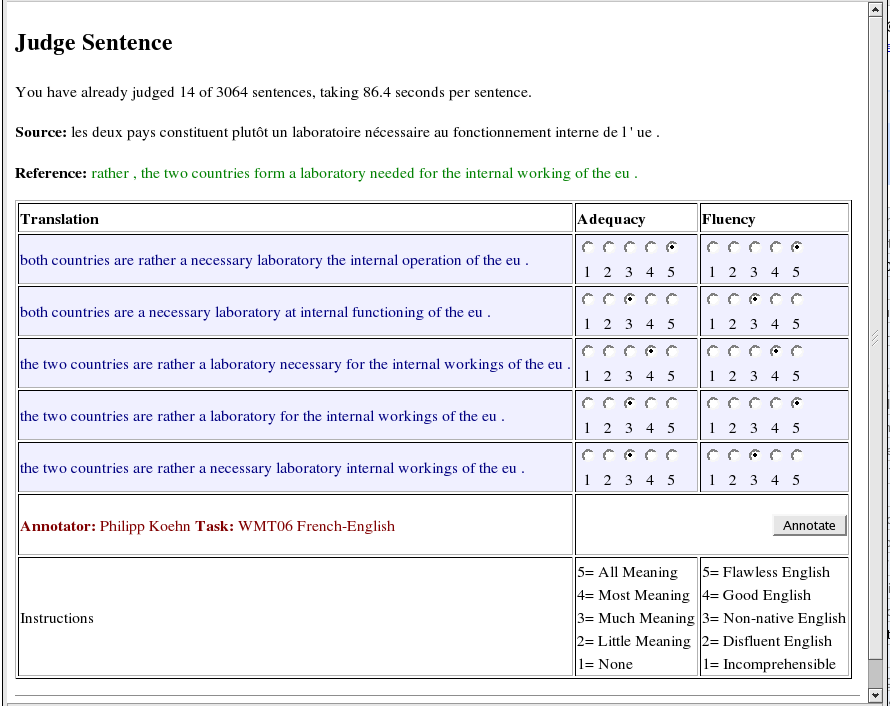• slow, expensive, subjective
• inter-annotator agreement (IAA) shows people agree more on fluency than on adequacy
• another option: is X better than Y? → higher IAA
• or time spent on post-editing
• or how much cost of translation is reduced

### Automatic translation evaluation

• disadvantages: do we really measure quality of translation?
• gold standard: manually prepared reference translations
• candidate $c$ is compared with $n$ reference translations $r_i$
where students are to assess their own exam: how they know
• various approaches: n-gram shared between $c$ and $r_i$, edit distance, …

### Recall and precision on words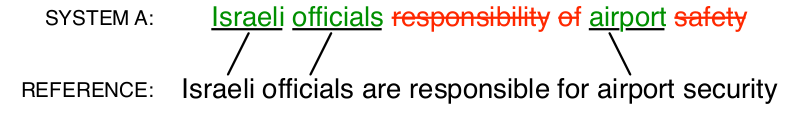$$\text{precision} = \frac{\text{correct}}{\text{output-length}} = \frac{3}{6} = 50%$$

$$\text{recall} = \frac{\text{correct}}{\text{reference-length}} = \frac{3}{7} = 43%$$

$$\text{f-score} = 2 \times \frac{\text{precision} \times \text{recall}}{\text{precision} + \text{recall}} = 2 \times \frac{.5 \times .43}{.5+.43} = 46%$$

### Recall and precision: shortcomings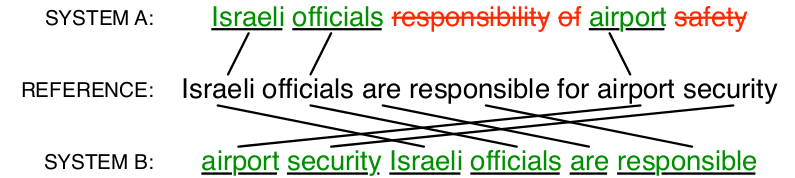metricssystem Asystem B
precision50%100%
recall43%100%
f-score46%100%

It does not capture wrong word order.

### BLEU

• standard metrics (2001)
• IBM, Papineni
• n-gram match between reference and candidate translations
• precision is calculated for 1-, 2- ,3- and 4-grams
• brevity penalty

$$\hbox{BLEU} = \min \left( 1,\frac{\text{output-length}}{\text{reference-length}} \right) ; \big( \prod_{i=1}^4 \text{precision}_i \big)^\frac{1}{4}$$

### BLEU: an example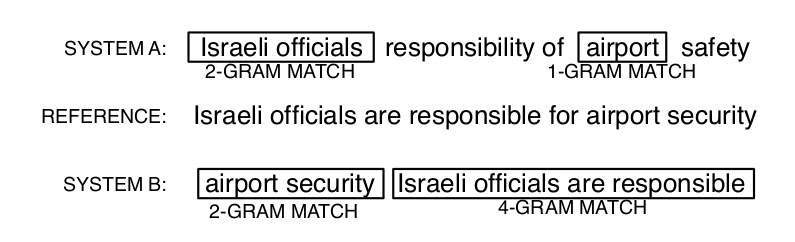metricssystem Asystem B
precision (1gram)3/66/6
precision (2gram)1/54/5
precision (3gram)0/42/4
precision (4gram)0/31/3
brevity penalty6/76/7
BLEU0%52%

### NIST

• NIST: National Institute of Standards and Technology
• weighted matches of n-grams (information value)
• very similar results as for BLEU (a variant)

### NEVA

• Ngram EVAluation
• BLEU score adapted for short sentences
• it takes into account synonyms (stylistic richness)

### WAFT

• Word Accuracy for Translation
• edit distance between $c$ and $r$

$\hbox{WAFT} = 1 - \frac{d + s + i}{max(l_r, l_c)}$

### TER

• Translation Edit Rate
• the least edit steps (deletion, insertion, swap, replacement)
• $r =$ dnes jsem si při fotbalu zlomil kotník
• $c =$ při fotbalu jsem si dnes zlomil kotník
• TER = ?

$$\hbox{TER} = \frac{\hbox{number of edits}}{\hbox{avg. number of ref. words}}$$

### HTER

• Human TER
• $r$ manually prepared and then TER is applied

### METEOR

• aligns hypotheses to one or more references
• exact, stem (morphology), synonym (WordNet), paraphrase matches
• various scores including WMT ranking and NIST adequacy
• extended support for English, Czech, German, French, Spanish, and Arabic.
• high correlation with human judgments

### Evaluation of evaluation metrics

Correlation of automatic evaluation with manual evaluation.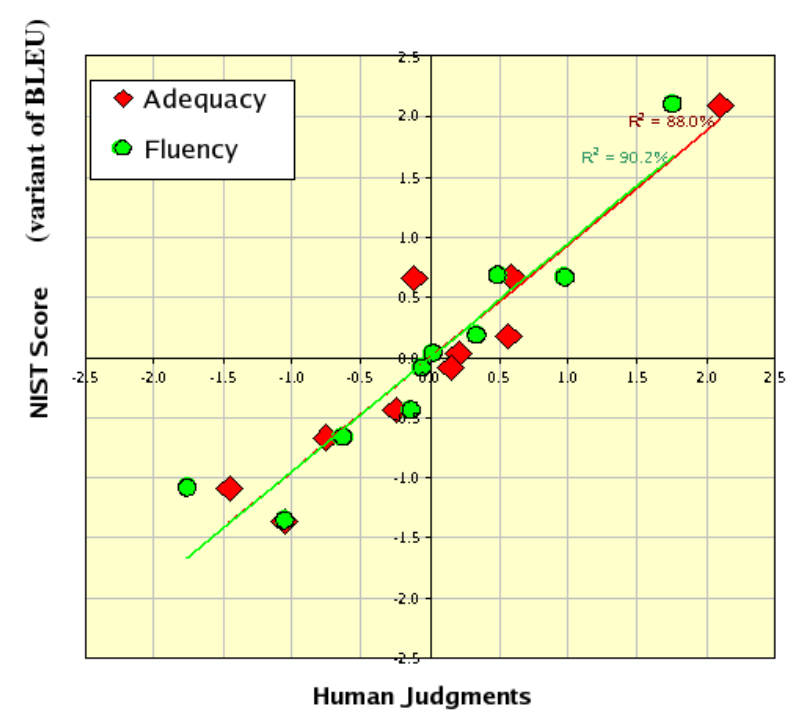### EuroMatrix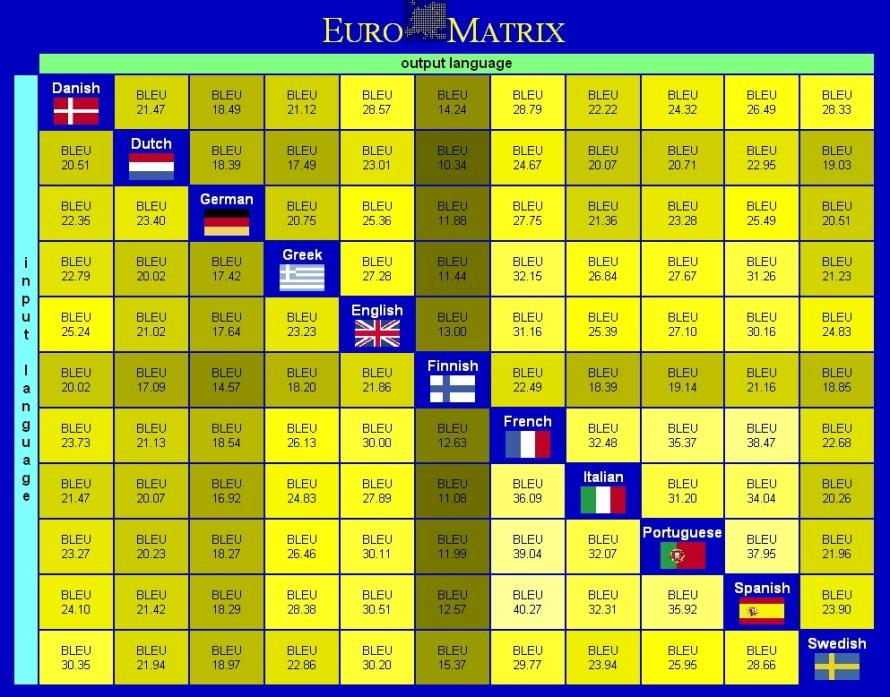### EuroMatrix II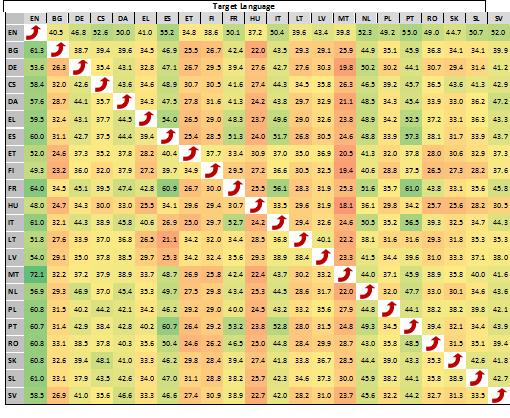### Round-trip translation

• a kind of evaluation
• DEMO

### Factored translation models I

• SMT models do not use linguistic knowledge
• lemmas, PoS, stems helps with data sparsity
• translation of vectors instead of words (tokens)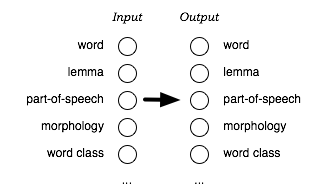• in standard SMT: dům and domy are independent tokens
• in FTM they share a lemma, PoS and morph. information
• lemma and morphologic information are translated separately
• in target language, appropriate wordform is then generated
• FMT in Moses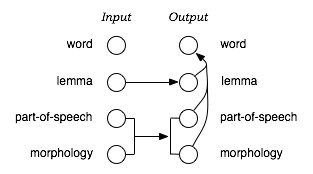### Tree-based translation models

• SMT translates word sequences
• many situations can be better explained with syntax:
moving verb around a sentence, grammar agreement at long distance, …
• → translation models based on syntactic trees
• for some language pairs it gives the best results

### Synchronous phrase grammar

• EN rule: NP → DET JJ NN
• DE rule: NP → DET NN JJ
• synchronous rule NP → DET$_1$ NN$_2$ JJ$_3$ | DET$_1$ JJ$_3$ NN$_2$
• final rule N → dům | house
• mixed rule N → la maison JJ$_1$ | the JJ$_1$ house

### Parallel tree-bank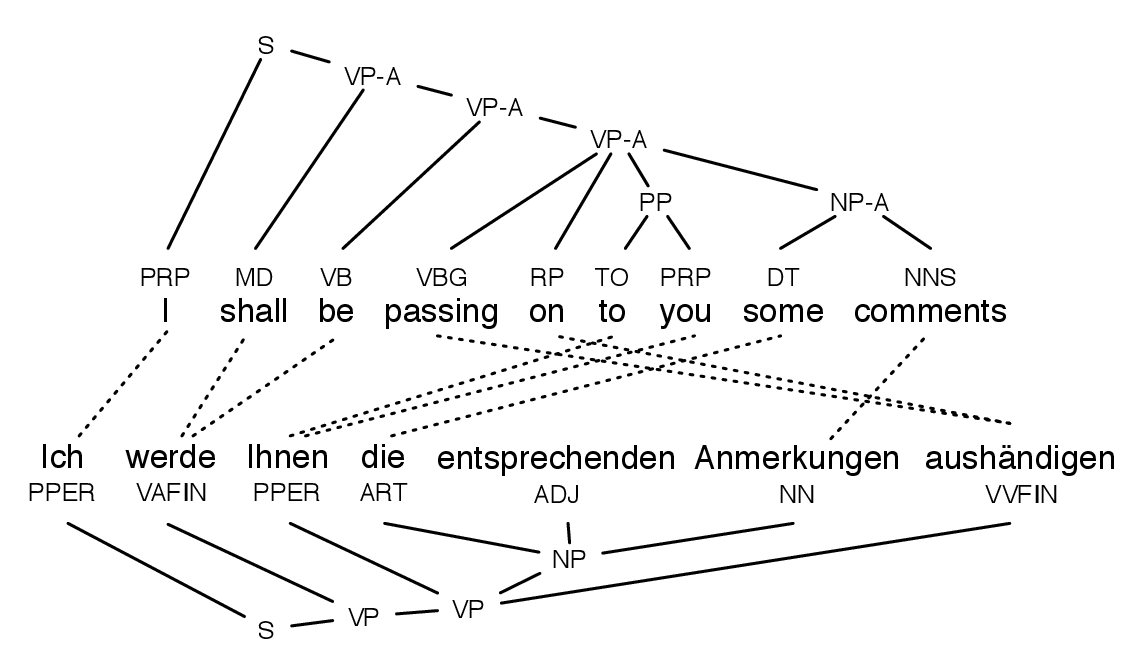### Syntactic rules extraction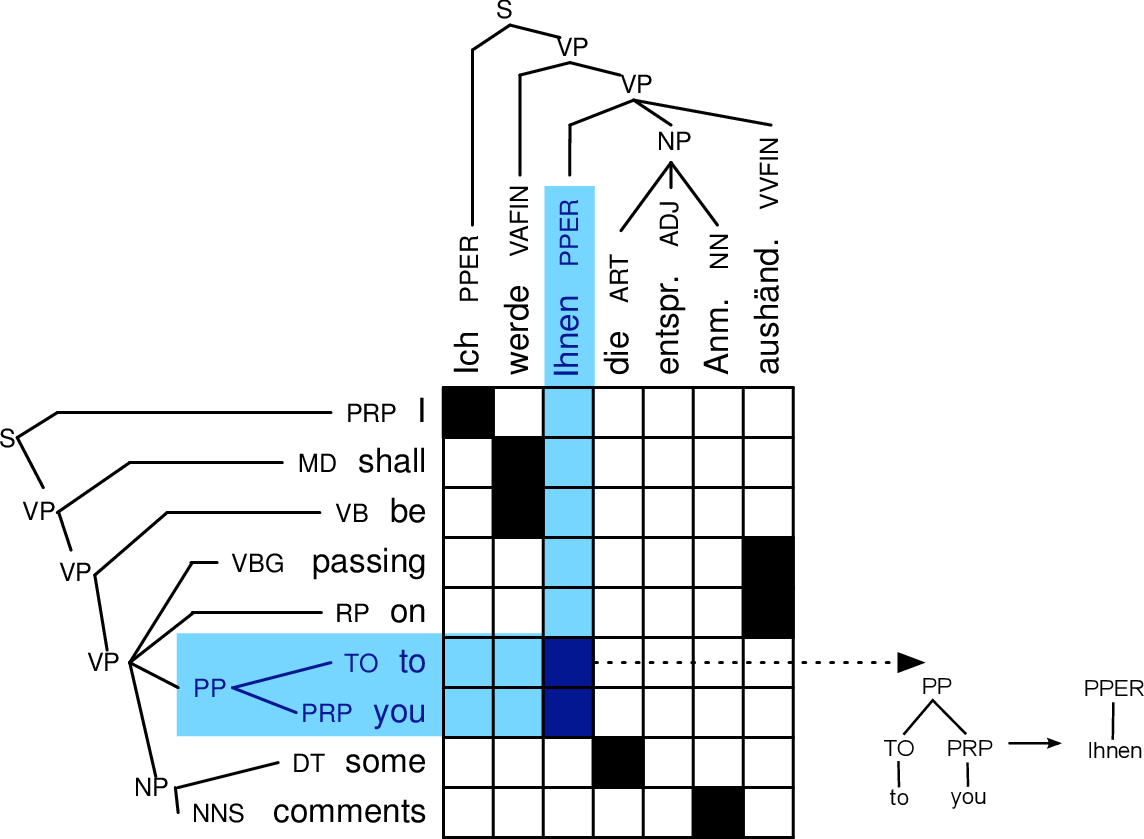### Hybrid systems of machine translation

• combination of rule-based and statistical systems
• rule-based translation with post-editing by SMT (e.g. smoothing with a LM)
• data preparaion for SMT based on rules, changing output of SMT based on rules

## Hybrid SMT+RBMT

• Chimera, UFAL
• TectoMT + Moses
• better than Google Translate (En-Cz)

### Computer-aided Translation

• CAT – computer-assisted (aided) translation
• out of score of pure MT
• tools belonging to CAT realm:
• spell checkers (typos): hunspell
• grammar checkers: Lingea Grammaticon
• electronic translation dictionaries: Metatrans
• corpus managers: Manatee/Bonito

### Translation memory

• DB of segments: titles, phrases, sent., terms, par.
• translated manually → translation units
• everything is translated only once
• cost reducing (repeated translation)
• majority is commercial
• translation units are know-how
• CAT suggests translations based on exact match
• vs. exact context match, fuzzy match
• combining with MT

### Questions: examples

• Enumerate at least 3 rule-based MT systems.
• What does abbreviation FAHQMT mean?
• What does IBM-2 model adds to IBM-1?
• Explain noisy channel principle with its formula.
• State at least 3 metrics for MT quality evaluation.
• State types of translation according to R. Jakobson.
• What does Sapir-Whorf hypothesis claim?
• Describe Georgetown experiment (facts).
• State at least 3 examples of morphologically rich languages (different language families).
• What is the advantage of systems with interlingua against transfer systems?
Draw a scheme of translations between 5 languages for these two types of systems.
• Give an example of a problematic string for tokenization (English, Czech).
• What is tagset, treebank, PoS tagging, WSD, FrameNet, gisting, sense granularity?
• What advantages does space-based meaning representation have?
• Which classes of WSD methods do we distinguish?
• Draw Vauquois’ triangle with SMT IBM-1 in it.
• Explain garden path phenomenon and come up with an example for Czech (or English) not used in slides.
• Draw dependency structure for sentence
Máma vidí malou Emu.
• Draw the scheme of SMT.
• Give at least 3 sources of parallel data.
• Explain Zipf’s law.
• Explain (using an example) Bayes’ rule (state its formula).
• What is the purpose of decoding algorithms?
• Write down the formula or describe with words Markov’s assumption.
• Examples of frequent 3-, 4-grams (Cz, En).
• We aim at low or high perplexity in language models?
• Describe IBM models (1–5) briefly.
• Draw word alignment matrix for sentences
I am very hungry.